The SAT Math section is notoriously algebra-heavy, and many of the more frequently occurring algebra questions involve quadratics. Quadratics appear somewhat less frequently on the ACT Math section, but having a strong understanding of how to work with these equations is still vital for success. Some students struggle with quadratics questions because they see the problems as far more complicated than they really are. In reality, most quadratics questions are designed to be solved in just a few simple steps.

Quadratic questions are easy to spot because there will always be a term in which the variable is squared.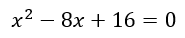The above example is ideal because there is no coefficient in front of the squared variable and all of the numbers are on one side of the equals sign. However, test questions aren’t usually presented in such a straightforward way.

First, the squared variable might not be by itself. Instead, it might have a coefficient attached to it. In these cases, it’s best to divide the whole equation by any common factors.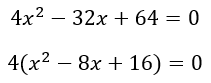Second, the equation may not equal zero at first. Make one side of the equation equal to zero as early in the solving process as possible.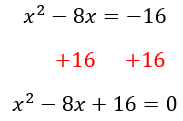Step 2: Orient yourself.

When it comes to quadratics, there are really only two directions you can travel. Either the question will provide you with an expanded equation that you need to factor or the question will provide you with factors that you need to expand. Identifying which direction to travel is half the battle.You should factor if a quadratic question gives you an equation and asks you to find any of the following:

• Solutions
• Roots
• Sum/difference/product/quotient of the solutions or roots
• Maximum or minimum

You should expand if the question gives you the solutions or roots and asks you to find one of these:

• An equation
• The value of a coefficient inside an equation

Step 3: Look for shortcuts.

One way to save some time on quadratic questions is to look out for perfect squares. On the non-calculator SAT Math in particular, many of the quadratic questions feature perfect square trinomials that follow very predictable patterns.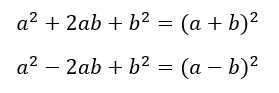Take the following equation, for example.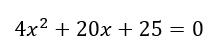The above equation includes perfect squares in the first and third terms.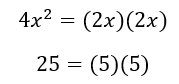You can therefore easily factor this equation.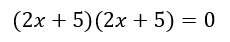Even when the given equation is not a perfect square, you can still use shortcuts to solve.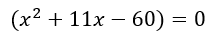Instead of reaching for a long and complicated formula to factor this equation, simply ask, “What factors of -60 add up to 11?” The answer is 15 and -4, so you can factor the equation as follows: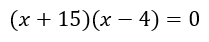A final shortcut to keep in mind pertains to the roots themselves. Some word problems describe a quadratic with only one solution or one root. In these cases, use the solution to create two matching binomials and expand from there.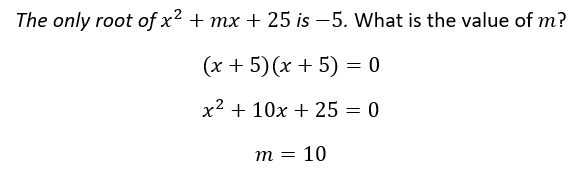Step 4: Answer their question, not yours.

Once you’ve taken the above steps, you’re ready to solve. Just be careful to answer exactly what is asked of you. Test writers are adept at creating answer choices that solve a portion of the equation but do not answer the question itself.

Try using a step-by-step approach to solve a typical SAT quadratic question.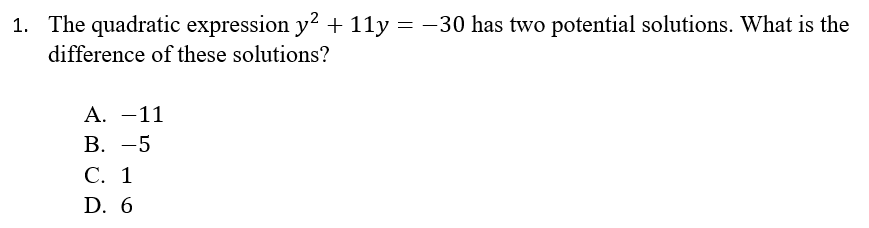First, simplify the equation by setting the equation equal to zero.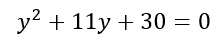Second, decide which direction to take your math. Will you factor or expand? The key word “solutions” appears in the question, so it looks like you’ll need to factor.

Third, check to see if you can take any shortcuts. The equation doesn’t contain any perfect squares, but there are only two factors of 30 add up to 11: five and six. You can therefore factor as follows: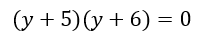Aha! Answer D shows the number 6. But wait! The question asked about the difference of the solutions. You must set each binomial equal to zero to solve for the solutions.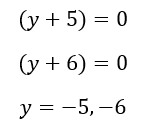Answer B shows one of these solutions. However, recall that the question asked for the difference of the two solutions, not the solutions themselves. Therefore, the correct answer is C.

As you can see, quadratic questions are more bark than bite. With careful attention to the wording of the question and practice with the various question types, these questions can become some of the easiest math questions to solve on test day.

The recommendations above are general suggestions. If you have specific questions, reach out to our experts here. We’re happy to help in any way we can.